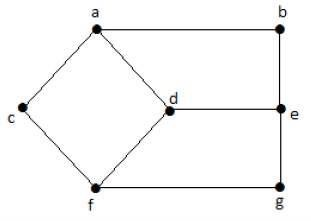# Graph Theory - Basic Properties

Graphs come with various properties which are used for characterization of graphs depending on their structures. These properties are defined in specific terms pertaining to the domain of graph theory. In this chapter, we will discuss a few basic properties that are common in all graphs.

## Distance between Two Vertices

It is number of edges in a shortest path between Vertex U and Vertex V. If there are multiple paths connecting two vertices, then the shortest path is considered as the distance between the two vertices.

### Notation − d(U,V)

There can be any number of paths present from one vertex to other. Among those, you need to choose only the shortest one.

Example

Take a look at the following graph −Here, the distance from vertex ‘d’ to vertex ‘e’ or simply ‘de’ is 1 as there is one edge between them. There are many paths from vertex ‘d’ to vertex ‘e’ −

• da, ab, be
• df, fg, ge
• de (It is considered for distance between the vertices)
• df, fc, ca, ab, be
• da, ac, cf, fg, ge

## Eccentricity of a Vertex

The maximum distance between a vertex to all other vertices is considered as the eccentricity of vertex.

### Notation − e(V)

The distance from a particular vertex to all other vertices in the graph is taken and among those distances, the eccentricity is the highest of distances.

Example

In the above graph, the eccentricity of ‘a’ is 3.

The distance from ‘a’ to ‘b’ is 1 (‘ab’),

from ‘a’ to ‘c’ is 1 (‘ac’),

from ‘a’ to ‘d’ is 1 (‘ad’),

from ‘a’ to ‘e’ is 2 (‘ab’-‘be’) or (‘ad’-‘de’),

from ‘a’ to ‘f’ is 2 (‘ac’-‘cf’) or (‘ad’-‘df’),

from ‘a’ to ‘g’ is 3 (‘ac’-‘cf’-‘fg’) or (‘ad’-‘df’-‘fg’).

So the eccentricity is 3, which is a maximum from vertex ‘a’ from the distance between ‘ag’ which is maximum.

In other words,

e(b) = 3

e(c) = 3

e(d) = 2

e(e) = 3

e(f) = 3

e(g) = 3

## Radius of a Connected Graph

The minimum eccentricity from all the vertices is considered as the radius of the Graph G. The minimum among all the maximum distances between a vertex to all other vertices is considered as the radius of the Graph G.

### Notation − r(G)

From all the eccentricities of the vertices in a graph, the radius of the connected graph is the minimum of all those eccentricities.

Example

In the above graph r(G) = 2, which is the minimum eccentricity for ‘d’.

## Diameter of a Graph

The maximum eccentricity from all the vertices is considered as the diameter of the Graph G. The maximum among all the distances between a vertex to all other vertices is considered as the diameter of the Graph G.

Notation − d(G) − From all the eccentricities of the vertices in a graph, the diameter of the connected graph is the maximum of all those eccentricities.

Example

In the above graph, d(G) = 3; which is the maximum eccentricity.

## Central Point

If the eccentricity of a graph is equal to its radius, then it is known as the central point of the graph. If

e(V) = r(V),

then ‘V’ is the central point of the Graph ’G’.

Example

In the example graph, ‘d’ is the central point of the graph.

e(d) = r(d) = 2

## Centre

The set of all central points of ‘G’ is called the centre of the Graph.

Example

In the example graph, {‘d’} is the centre of the Graph.

## Circumference

The number of edges in the longest cycle of ‘G’ is called as the circumference of ‘G’.

Example

In the example graph, the circumference is 6, which we derived from the longest cycle a-c-f-g-e-b-a or a-c-f-d-e-b-a.

## Girth

The number of edges in the shortest cycle of ‘G’ is called its Girth.

Notation: g(G).

Example − In the example graph, the Girth of the graph is 4, which we derived from the shortest cycle a-c-f-d-a or d-f-g-e-d or a-b-e-d-a.

## Sum of Degrees of Vertices Theorem

If G = (V, E) be a non-directed graph with vertices V = {V1, V2,…Vn} then

n Σ i=1 deg(Vi) = 2|E|

Corollary 1

If G = (V, E) be a directed graph with vertices V = {V1, V2,…Vn}, then

n Σ i=1 deg+(Vi) = |E| = n Σ i=1 deg−(Vi)

Corollary 2

In any non-directed graph, the number of vertices with Odd degree is Even.

Corollary 3

In a non-directed graph, if the degree of each vertex is k, then

k|V| = 2|E|

Corollary 4

In a non-directed graph, if the degree of each vertex is at least k, then

k|V| ≤ 2|E|

| Corollary 5

In a non-directed graph, if the degree of each vertex is at most k, then

k|V| ≥ 2|E|